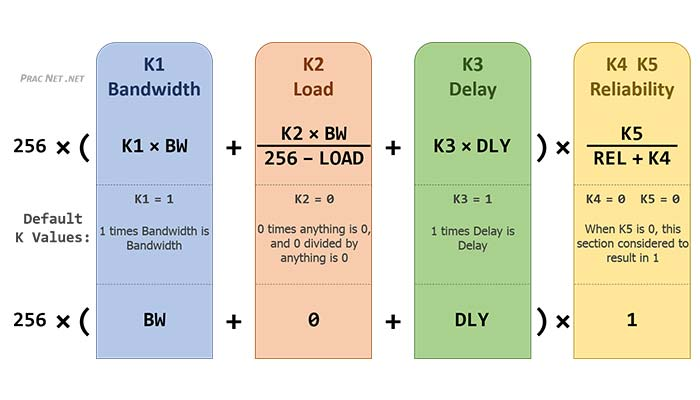(Enhanced Interior Gateway Routing Protocol) EIGRP Metric Calculation Formula is used to find the matric value of the route. EIGRP uses the matric formula to find the easiest route from all the routes. Using the EIGRP metric formula we can find the best route by finding the lowest cost of the path, which provides the best route. To know more about EIGRP Metric Calculation Formula, keep reading this article till the end.

The working principle is to reach the destination there will be multiple paths and by calculating the formula we can find the cost of each route and comparing all the cost each other we could find the lowest path which is then considered as the best path and the route will be placed in the routing table and other routes to the topology table.

METRIC CALCULATION

Five metrics are used by EIGRP, they are

• K1-bandwidth (slowest outgoing interface bandwidth measured in Kbits)
• K3-delay (sum of delay in the path)
• K4-reliability (average reliability of path)
• K5-MTU (interface min MTU)

EIGRP supports multiple inputs to the equation and they are by default EIGRP supports bandwidth and delay. EIGRP also supports the load and reliability along with the default inputs. MTU (maximum transmission unit) is also supported which includes the largest IP packet used in the route. The main point to understand is that during the calculation EIGRP does not use MTU.Here the bandwidth and delay are used default by EIGRP, therefore the value of metrics can be used as K1=1 and K2=0.

As mentioned above, term bandwidth represents the lowest bandwidth in kilobits per second. The delay value used is the total of all delay values in the route and is measured in “tens of microseconds”. Using these two inputs the easiest route can be found by EIGRP.

BANDWIDTH (K1)

It is the static value that only changes when changes to routes are done. Changes can be done by upgrading links or changing cables. EIGRP considers only the lowest bandwidth among others.

The values of load can be changed frequently based on bandwidth and rate of packet. Two metric counters are used here, Txload and Reload for outgoing and ingoing traffic. Maximum Txload values are used for metric calculation if K2 is enabled.

DELAY (K3)

Measures the maximum time taken to complete interface by packet. A sum of delay is used for calculation. It is a static value and by using the delay command we can override it. And are measured in tens of microseconds.

RELIABILITY (K4)

Here successful frames are compared with all frames and if reliability is 100% which means that received frames are good. Reliability is expressed in a fraction.

MTU (K5)

The maximum transmission unit is the expanded form of MTU. It is used to balance the cost of paths. If the allowed path cost will be set as 3 and it exceeds 4 to balance it, it is used.